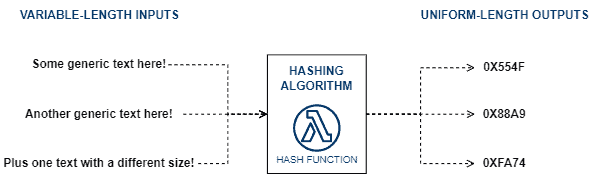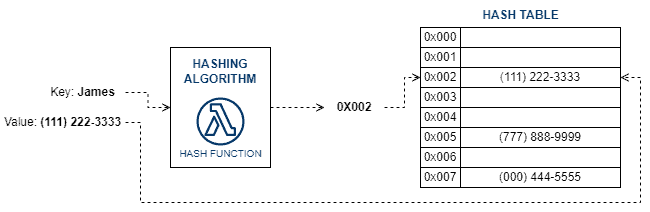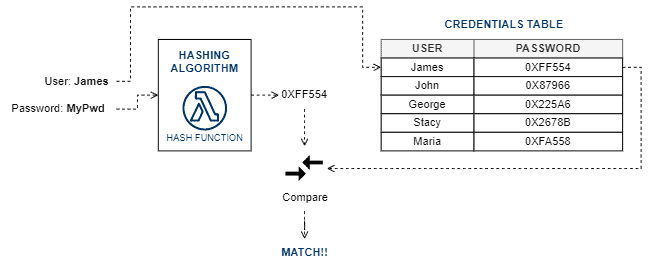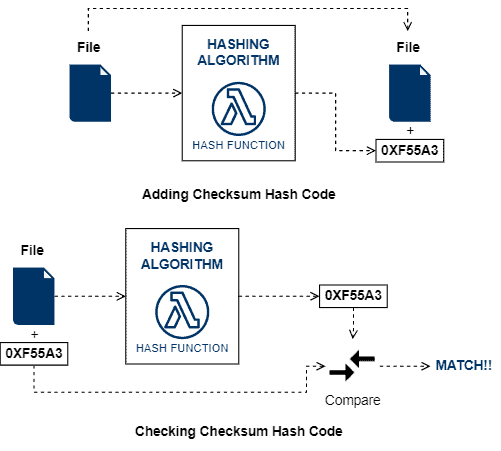## 1. Introduction

In this tutorial, we’ll study hash codes and checksums. Hash codes and checksums employ pretty similar processes during their creation. Due to this reason, they get confused with each other several times. But, despite their processes’ similarity, hash codes and checksums have peculiarities that make each more appropriate for different scenarios, as we’ll see in the following sections.

In this way, the second section presents some background concepts regarding both hash codes and checksums. So, the third section particularly discusses hash codes, while the fourth section focuses on checksums. Thus, the fifth section summarizes and compares both techniques, and the sixth section concludes the tutorial.

## 2. Background Concepts

First, we need to state that checksums are hash codes. Actually, we can see a hash code as a broad concept with multiple applications. So, let’s talk about the general idea and purpose of hashes initially to then focus on their applications.

The main idea behind hashing processes is transforming a bunch of data of any size into a code with a predefined size.

Hash methods employ hashing functions to do the data transforming process. So, by processing given data, the hash function generates a hash code (sometimes also called hash value, digest, or simply hash).

The following image depicts, in a high level of abstraction, the process of creating hash codes:Furthermore, it is relevant to highlight that hash codes are, by definition, irreversible. It means that hashing is a one-way process: they generate a code for a given data. However, they can not generate the original data from a hash code.

The presented characteristics of hashing methods made them suitable to tackle several challenges. Among the most popular facets of hashing are:

• Hash Table: a structure tailored for fast searching stored data
• Cryptographic Hash: security-based hashing functions which make it almost impossible to reverse a code to the original data (the one-way characteristic is pretty relevant here)
• Checksum: integrity-based hashing functions that aim to verify if a given data is the same as the one that generated a provided hash code

Next, we’ll concentrate on studying hashes as data structures and cryptographic strategies. So, we’ll investigate hashes as integrity functions in a section dedicated to checksums.

## 3. Hash Codes (General Uses)

As we previously stated, hash code is a broad concept. It means that we can adapt the hash function to generate codes according to our objectives, observing some desired characteristics.

In this context, two popular uses of hash codes are to build data structures called hash tables and to create one-way cryptographic codes. In the following subsections, we’ll study a little bit about each one of these uses.

### 3.1. Hash Tables

Hash tables are data structures that track given values with particular keys. So, in short, we can say that hash tables are key-based structures.

From an implementation point of view, the keys of a hash table have the same data type and length. However, from the user’s point of view, the keys can have different data types and lengths.

So, to conciliate the user requirements and the implementation necessities, a hash function is used to translate heterogeneous keys to a standard key format.

The following image depicts the storing generic data process in a hash table:In this case, the hash function developers are mainly concerned about avoiding hash collisions (multiple different keys that generate the same hash code).

Of course, we can solve collision scenarios with support techniques. But, these techniques increase the complexity of accessing and maintaining a hash table.

### 3.2. Cryptographic Hash

Cryptographic hashing is another pretty relevant use of hash codes. The main idea here is to create a hash code to encrypt and securely store sensitive data.

So, since we have one-way functions for hashing, it should be impossible (or almost impossible) to recover plain sensitive data from its hash.

But, given some plain data, we can define its hash code with the used cryptographic function, thus comparing the results with the previously generated hashes and looking for a match. So, if a pair of hash codes match, there is sufficient evidence that the same plain data created both codes.

Due to the described features, cryptographic hashing is frequently employed to store passwords.

The following image depicts the previously described process:In cryptographic hashing, the most relevant characteristic is the one-way function. In this way, we aim to keep the sensitive plain data safe even if their hash codes leak for some reason.

## 4. Checksum

Checksums are also hash codes. But, different from the use cases discussed in the last section, checksums are mainly focused on checking data integrity.

We can understand checksums as a fingerprint of some data. Thus, once provided the original data, we can take its fingerprint and compare it to an also provided one. If the fingerprints (checksums) match, we can say with high reliability that the data integrity is not compromised.

In this way, good hashing functions to checksums must be able to change their generated hash code given minimal changes in the input data. For instance, if we calculate the checksum for a bunch of data, change a single bit in the data, and recalculate the checksum, the results should be different.

We can employ checksums in multiple contexts, from the most traditional integrity check of stored data to error detection in networking transmissions and as part of a digital signature.

Since the same checksumming process can be executed many times in, for instance, the same data transmission through the network, the algorithms should be fast to compute and compare the hash codes.

The following image depicts the process of checking the integrity of a file through checksum:Finally, we can use several hashing algorithms to implement checksumming processes, such as:

• MD5: an algorithm that computes 128-bit hash codes appropriate to check data integrity. The RFC1321 describes MD5
• SHA-1: a strategy that generates a 160-bit hash code that we can use for checksumming. The RFC3174 describes SHA1

## 5. Systematic Summary

Hash codes became a crucial resource in computing. The possibility to map variable-length data to uniform length codes found several applications in multiple virtual world scenarios.

So, initially, we should note that hash codes are a broad concept, and for each application of them, we will have particular requirements and characteristics.

In such a way, we can understand checksums as one (and an important one) application of the hash code concept. Checksums consist of a uniform-length code calculated from generic data to check its integrity.

The main requirement differentiating checksums from other hash code applications, such as hash tables and cryptographic hashes, is that they should be fast to calculate. It occurs because the data integrity is typically tested several times during, for example, its transmission through the network.

The following table correlates generic hash codes concepts with their specific application for data integrity testing through checksums:## 6. Conclusion

In this tutorial, we studied hash codes and how they are related to checksums. At first, we reviewed background concepts about hashing. Thus, we investigated some general use cases of hash codes. Next, we deep-dive into checksumming, understanding how hash codes fit it. Finally, we systematically summarized the concepts of hash codes and checksums.

We can conclude that hash codes are necessary for several areas, from programming (with hash-based data structures) to security (with cryptographic hashes). Checksum, in turn, is another critical application of hash codes, enabling us to check data integrity in many contexts, such as when transmitting or storing them.